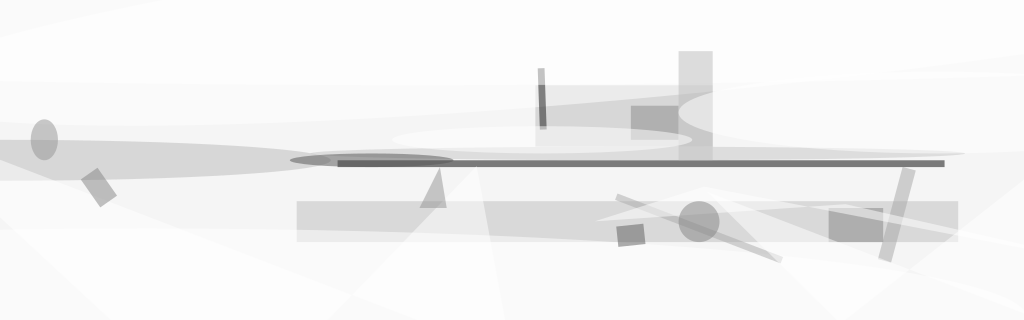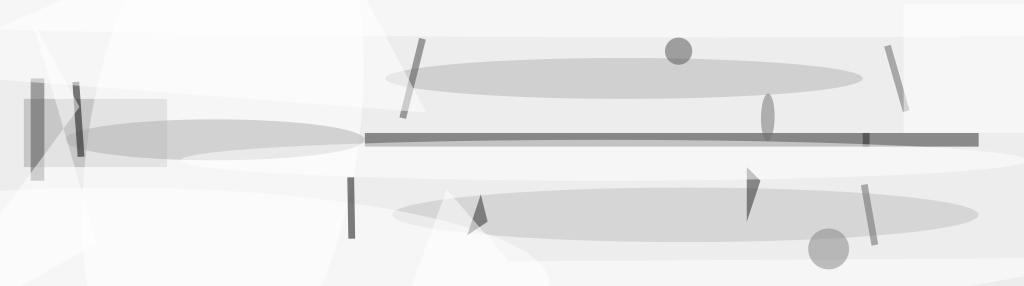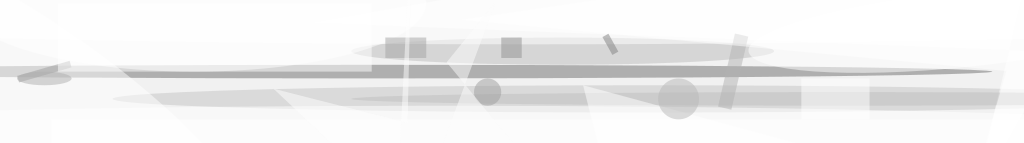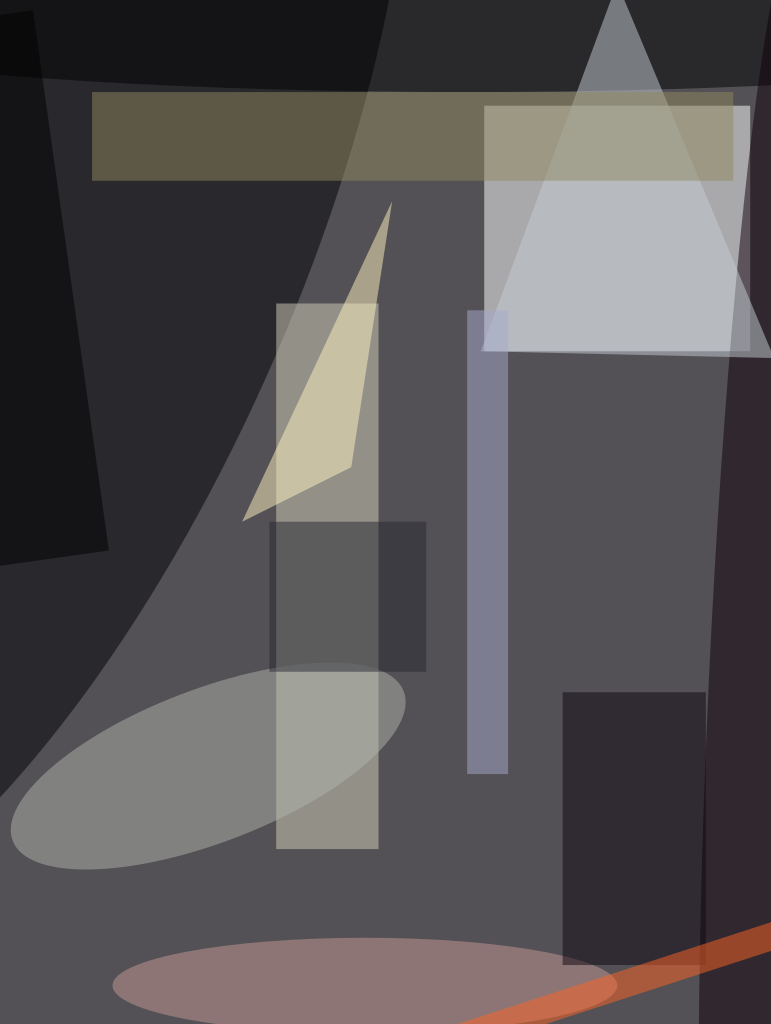Performing closed-loop control of hydraulic servo systems is often more challenging than controlling servomotor systems. The main reason is that hydraulic systems use compressible oil to move the actuator. Because of this, a hydraulic system can be modeled as a mass between two springs, where the piston and the load is the mass, and the oil on both sides of the piston represents the two springs. In contrast, servomotor systems are easier to control because there is basically only the inertia of the motor and the connected load to be dealt with.

There is enough difference between the two types of systems such that a strategy for controlling one is not going to be optimal for the other. Servomotor systems can be controlled very well with PID (proportional integral derivative) control loop gains plus velocity and acceleration feed forward gains, but a simple PID loop, even with feed forward components, cannot always control a hydraulic system optimally.

Some of the more challenging hydraulic systems are underdamped (i.e., could be prone to oscillations), so a higherorder derivative gain often needs to be used to add electronic damping. To control a second-order underdamped system well requires knowing what the system’s closed-loop transfer function will look like, which, for controls engineers, takes us into s-plane analysis in control theory. The hydraulic actuator and load will have two poles in the s-plane that are underdamped. There is another pole that must be added to integrate the velocity of the actuator to position. A fourth pole is added by the controller’s integrator. For a quick response to errors and to reduce oscillations, it is best to move all the open-loop poles closer to the negative real axis in the s-plane and away from the origin.

#### The Problem

It can be proven symbolically that using only PI or PID control has severe limitations as to where the closed-loop poles can be located. First, it is impossible to place all the closed-loop poles on the s-plane’s negative real axis except at one point, and that is at −ζωn/2 where ζ is the damping factor (dependent on the system configuration) and ωn is the natural frequency of oscillation of the actuator and the load (see Figure 1a). In all other cases, there will be complex pairs of poles that will result in oscillations. The response will also suffer because no matter how much the control person twiddles with the P, I, and D gains, it is impossible to improve the response by moving all the closed-loop poles to the left or more negative than −ζωn/2. It is possible to move some of the poles to the left of −ζωn/2 but as one moves one or two of the closed-loop poles to the left, there will be one or two closed-loop poles that move to the right or closer to the origin. The poles closer to the origin will be dominant poles and will limit the response. What this means is that there is little the control person can do to increase response in an underdamped system if all that he or she has is a conventional PID loop controller. The control person is limited by the damping factor and the natural frequency, and these attributes are determined by the hydraulic and mechanical design.

However, the four closed-loop poles can be moved to the left of −ζωn/2 if a second derivative gain is added to the mix (see Figure 1b). When four gains are used — which correspond to an integrator, proportional, derivative, and a second derivative gain in the control loop equation — it is possible to place the four closed-loop poles just about anywhere within reason, but the goal is to move the closed-loop poles to the left or more negative of −ζωn/2 and keep them close to the negative real axis to minimize oscillations. Most people think that a PID controller with feed forwards is enough, and it may be when the system is stiff enough, but when the damping factor and natural frequency are low, then a second derivative gain may be necessary.

The following analysis shows that it is impossible to move all the poles left of −ζωn/2 in the s-plane to achieve better performance if only a PID controller is available. In the case of a hydraulic system, a simplified transfer function for a hydraulic cylinder in position control mode isThere are two complex poles (s2) that implement the mass between two springs, and one pole (s) at the origin that integrates velocity to yield the position value.

The transfer function for a PID controller iswhere Ki is the integrator gain factor, Kp is the proportional gain, and Kd is the derivative gain.

The transfer function for a closed loop system isAfter substituting for the controller and actuator transfer function and simplifying, the resulting closed-loop transfer function isThe denominator is the characteristic equation for the closed-loop system. Notice that there isn’t a controller gain for the s3 term. The lack of gain for the s3 term is what limits the closed-loop response. Since the characteristic equation is a fourth order polynomial, there are four poles. To place the four poles, there needs to be four equations and four unknowns. There are four unknowns in the controller equation. The first three unknowns are Ki, Kp, and Kd (which correspond to the integral, proportional, and derivative gains in the control loop), and λ must be added for the fourth unknown. To generate the four equations, a desired closedloop characteristic equation is needed. To make it simple, the desired closed-loop characteristic equation is (s+λ)4 so there will be four poles at −λ. This should result in a critically damped response to any errors because there are no imaginary or complex terms. Four equations can be generated by equating the coefficients for each of the powers of s.

The equation generated from the s0 coefficients is λ4=Κі∙Κ∙ω n 2 . It is easy to solve for the integrator gain Ki if you know K and ωn for s3 the equation is 4λ =2ζω which reduces to λ=ζω/2 and the characteristic equation is (s+ζ∙ω/2)4. Different desired characteristic equations can be tried but none of the options will allow any of the poles to be moved to the right of −ζω/2 without moving others to the left, closer to the origin, resulting in an even slower response.

A PI controller has a similar problem as the PID controller. The poles are limited by −ζω/2, but since there are only two gains, only two poles can be real. The other two poles will be a complex pair that will allow oscillations. It is possible to move two poles farther away from the imaginary axis, but the other two poles will move closer to the origin as a result, and slow the response.

#### The Solution

If a second derivative gain is used, then the closed loop transfer function isso there is a controller gain K2 for s3. The four controller gains can be found using the four equations; λ is no longer limited by the hydraulics or mechanics. Now the four closed-loop poles can be moved to the left of −ζω/2 of by increasing λ to increase the response of the system.

Per the above conclusions, if the hydraulic motion controller being used for a particular application is only capable of implementing a simple PID algorithm for closed-loop control, the response or bandwidth of the system will be limited by the system’s damping factor and natural frequency. Only the mechanical and hydraulic designers have any control over these values and the resulting limitations in bandwidth, and in most practical cases, the natural frequency of the system is never calculated during the design and specification process. Calculating or estimating the damping factor is more complicated and usually can only be estimated empirically, while increasing the natural frequency is accomplished by increasing the diameter of the cylinder. Increasing the cylinder size results in extra costs for bigger valves and hydraulic power units, while increasing the damping factor is accomplished by adding friction or leakage that increase operating costs and waste energy.

The most economical way of increasing a motion system’s response or bandwidth is to use a motion controller that has been designed to incorporate the second derivative gain (DDiff. Gain in Figure 2) such as those shown in Figure 3.